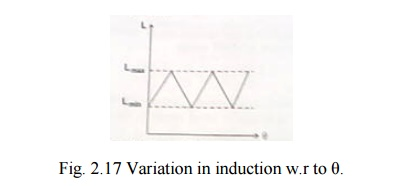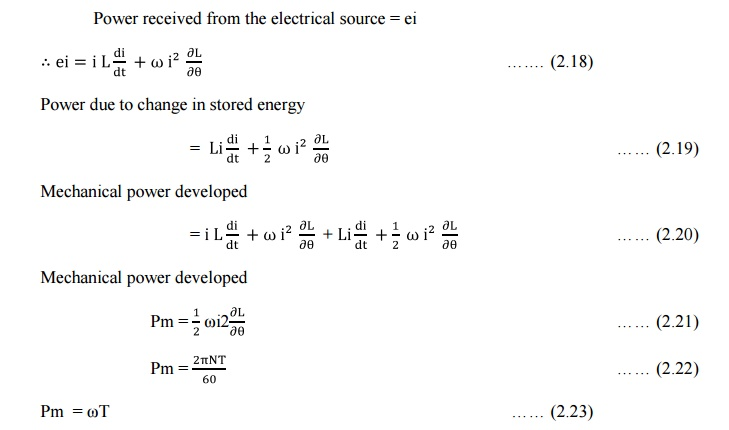Home | | Special Electrical Machines | Theory of Torque Prediction

# Theory of Torque Prediction

According to Faradays laws of electromagnetic induction

THEORY OF TORQUE PREDICTION

According to Faradays laws of electromagnetic inductionIf the reluctance of magnetic circuit can be varied, inductance L and the flux linkages λ can also be varied.

Consider a magnetic circuit as shown in fig. 2.29.The stator consists magnetic core with two pole arrangement. Stator core carries a coil. Rotor is also made up of ferrous material. The motor core is similar to a salient pole machine. Let the angle between the axis of stator pole and rotor pole be θ. let the angular displacement be illustrated using fig. 2.29 (a, b and c).

Case 1: θ = 0

As shown in fig. 2.29 (a) the air gap between the stator and rotor is very very small. Thereby the reluctance of the magnetic path is least. Due to minimum reluctance, the inductance of the circuit is minimum. Let it be Lmax

Case 2 : θ = 450

As shown in fig. 2.29(b) in this only a portion of rotor poles cover the stator poles. Therefore reluctance of the magnetic path is more than that of case 1.due to which the inductance becomes less than Lmax .

Case 3: θ = 900

As shown in fig. 2.29(c) the air gap between the stator poles has maximum value. Thereby reluctance has a value yielding minimum inductance. Let it be  Lmax.

Variation in inductance with respect to the angle between the stator and rotor poles is shown in fig. 2.30.Derivation for reluctance torque

As per faradays law of electromagnetic induction an emf induced in an electric circuit when there exists a change in flux linkages.If the direction of current I is opposite to that of e, then the electric power is transferred from the source to the inductor. On the other hand, if the direction of current I is same as that of e, then the source gets the electrical power from the inductor.

On the basis of magnetic circuit/field theory it is known that the stored energy in a magnetic field.The rate of change of energy transfer due to variation in stored energy or power due to variation in stored energy.Mechanical power developed/consumed = power received from the electrical source – power due to change in stored energy in the inductor

Power received from the electrical source = ei*      Torque is proportional to i2 : Therefore it does not depend upon the direction of the current.

Study Material, Lecturing Notes, Assignment, Reference, Wiki description explanation, brief detail
Special Electrical Machines : Stepping Motor : Theory of Torque Prediction |